# Home Loan Manager - User Manual

## Interest Calculations

When you first set up an account in Home Loan Manager Pro you may need to fine-tune the interest calculation settings to match those used by your lender. This help topic is the best place to start if the interest checker report is displaying errors:

In order to check the accuracy of the interest charges on your loans, Home Loan Manager Pro has to calculate its own estimate for every one of your interest transactions. To do this, the interest checker report has to:

1. Identify all the interest charges on your statements.
2. Calculate the date range each charge applied to.
3. Find all the changes to the balances in the date range.
4. Find all interest rate changes in the date range.
5. Calculate the interest amount.

The following is a quick example of how the interest checker report processes an interest transaction (along with tips on the information it uses):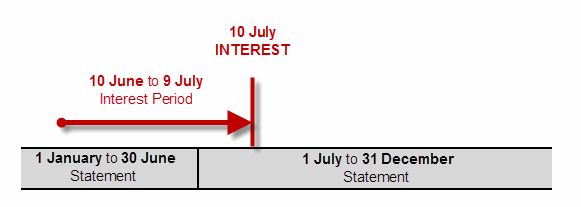1. The software finds an interest charge on the 10th of July.

Tip - Home Loan Manager Pro requires that you assign the category "INTEREST - Periodic Interest" to all you interest charge transactions.

2. It calculates the interest period to be the 10th of June to the 9th of July.

Tip - Home Loan Manager Pro automatically calculates this date range in one of two ways (1) using the transaction date and then subtracting the default value in the Days Calculated Up To field on the account, or (2) using the Days Interest Calculated Up To date on the transaction if you have manually set it.

3. It looks for all balance changes in this interest period.

Tip - Home Loan Manager Pro uses a combination of the statement opening balances and the transaction amounts to calculate daily balances.

4. It looks for the interest rate changes in the interest period.

Tip - Home Loan Manager Pro uses the interest rates you have entered for the account, and converts them into values that can be used in the interest calculations using the value in the Days In Year field on the account.

5. It estimates the interest based on the above information.

Tip - Home Loan Manager Pro uses the standard internationally accepted formula for calculating interest on the outstanding daily balance, in arrears.

If the interest checker can't calculate the interest, or it calculates the interest and it doesn't match the amount charged by your lender, it will display an error. The errors displayed in the interest checker report will be one of three types.

Interest Errors
The estimated interest has been calculated, however it doesn't match the amount your lender has charged you. This could be due to incorrect settings in the software, or it could be due to a lender error.

Tip - These errors will be displayed under the Difference column in the report.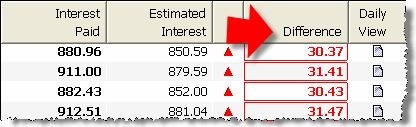Missing or Incorrect Information
The report cannot calculate an estimate as you have not entered all the information required.

Tip - These errors will be displayed under the Interest Paid column in the report.Rounding Errors
The estimated interest has been calculated, and there is a very small difference between it and the amount you have been charged by your lender.

Tip - These errors will be displayed under the Difference column in the report.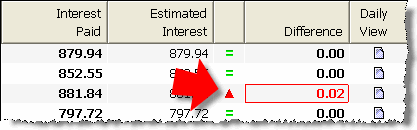### 3. Interest Errors

The first time you run the interest checker report you may find errors in most of your interest transactions. Clicking on an interest error in the report will open a window displaying the key information used to calculate the estimate.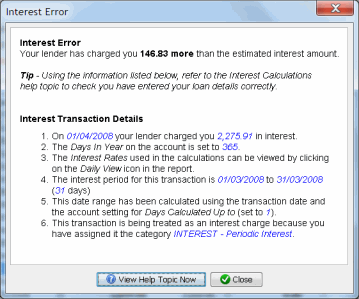The following list will help you check that you have entered your information correctly and let you distinguish between small fine-tunning issues, and lender mistakes:

1. Setting the Days In Year Account Value
2. Entering Interest Rates
3. Calculating the Interest Period Date Range
4. Using the INTEREST Category
5. Setting the Interest Transaction Date
6. Other Interest Errors

### 4. Setting the Days In Year Account Value

Tip - Check that you have entered the correct value in the Days in Year field on the account (most common value is 365).

The Days In Year on the account is used to convert the yearly rates provided by your lender into a value that can be used in the daily interest calculations. If you get this value wrong it will impact most of interest transactions.

One common mistake when entering your loan details is to change the default value from 365 to Actual. If you set the value to Actual the software will use 366 days for leap years, and 365 days for non-leap years.

Lenders generally use a fixed 365 days, even on leap years. You can check the value your lender uses by looking at your loan contract or accompanying documents your lender has provided you with explaining you interest charges.

Therefore if you have selected Actual, and the interest checker report is showing a small error on every interest charge for leap years (eg 1996, 2000, 2004, 2008, etc) then you may need to change the value back to 365.

Example - Leap Year Problem

The following example is from the Home Loan in sample workbook, where we have changed the Days In Year field from the correct value of 365 to an incorrect value of Actual. Errors are being displayed in the leap year.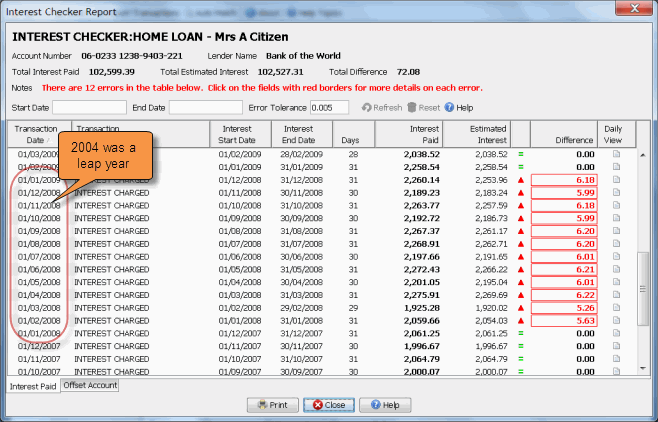### 5. Entering Interest Rates

Tip - Check that you have entered all the interest rate changes for the account. If you miss an interest rate change, or you enter the wrong date or rate, then all interest calculations from that point forward will be incorrect.

Each time your interest rate changes, your lender will provide you with a new interest rate along with the date they started using the rate. Make sure you have created one Interest Rate record for each interest rate change on the account, and check that you have entered the correct interest Start Date and Rate.

Example - Missing Interest Rates

The following example is from the sample workbook, where we have deleted the interest rate change on the 1st of February 2004 that was for 7.25%. Errors are being displayed from February 2004 onwards.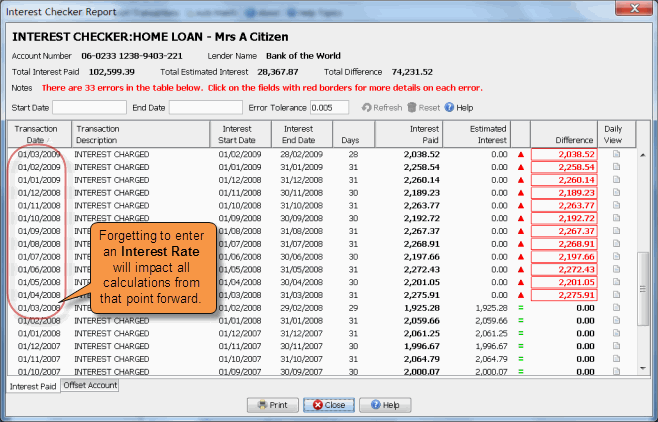### 6. Calculating the Interest Period Date Range

Tip - If the Days Calculated Up to field on the account contains the wrong value then every interest transaction in the interest checker report will contain an error. Check that the report is displaying the correct date range for each transaction and that the days in the interest period looks correct (eg if you are charged interest monthly then you would expect a days value between 28 and 31).

When Home Loan Manager Pro finds an interest transaction on your statement, it has to calculate the interest period the transaction relates to:

1. To calculate the end date it uses the date on your interest transaction and then subtracts the default number of days you have set up in the Interest Calculated Up to ... field on the account.
2. To calculate the start date it uses the end date of the previous interest period (or the loan start date if it is the first interest transaction on the account).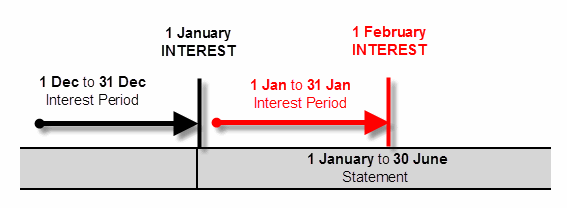By default the Days Calculated Up To value is set to one, so when Home Loan Manager Pro finds an interest transaction it will use the day before the transaction as the end date. For example, for an interest transaction on the 1st of February it will subtract 1 day from the transaction date and use the end date of 31 January.

If you lender charges interest on the same day as they calculate the interest period, then you will need to change the account setting to zero.

If your lender doesn't follow a set pattern, or they process a transaction late one month, then you can edit the individual interest transactions and manually specify the required end date in the field Date Interest Calculated Up To. When you set the value on a transaction Home Loan Manager Pro will ignore the default value on the account.

Example - wrong Days Calculated Up To value on the account

The following example is from the Home Loan in the sample workbook, where we have changed the days calculated up to value from the correct value of one to the incorrect value of zero. Errors are being displayed on every transaction.### 7. Using the INTEREST Category

Tip - Check that each interest transaction has been allocated an INTEREST category. Check that no other transaction has been allocated an INTEREST category.

The Interest Checker Report will only process transactions that have been allocated a category of type INTEREST. If the report is missing an interest transaction, then close the report, look for the missing transaction in the account, and assign it an INTEREST category

If the report is displaying transaction that are not interest charges, close the report, look for the transaction in the account, and change their category to something other than INTEREST

Example - missing INTEREST transaction

The following example is from Home Loan account in the sample workbook, where we have changed the category on the interest transaction dated 1st April 2007 from the correct value of "INTEREST - Periodic Interest" to an incorrect value of "FEE - Other". NOTE: These errors are fairly easy to find as shown below.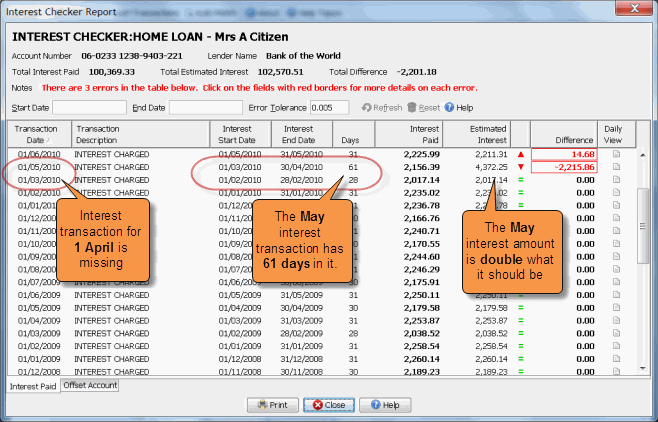### 8. Setting the Interest Transaction Date

Tip - Check that you have entered the interest transaction date correctly.

If you enter the wrong date on an interest transaction then it will result in two errors on the interest checker report.

For example, if you accidentally enter the 2nd of January instead of the 1st of January, it will cause create an error in both the January and February interest calculations, as the interest period shifts by one day.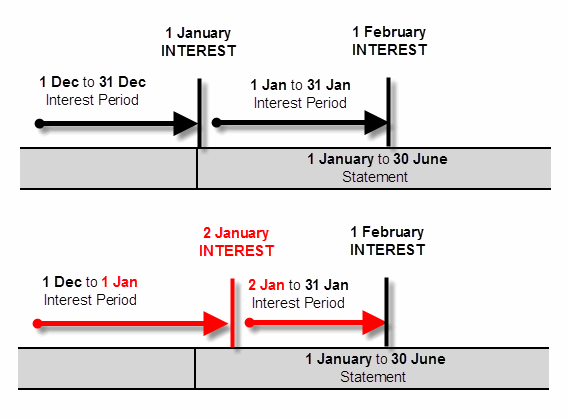By entering the wrong interest date, the January interest estimate will contain an extra day, and the February estimate will be missing a day.

Example - Incorrect Interest Transaction date

The following example is from Home Loan account in the sample workbook, where we have changed the date on the interest transaction from the correct value of 1 January 2007 to an incorrect vale of 2 January 2007. NOTE: These errors are fairly easy to find as shown below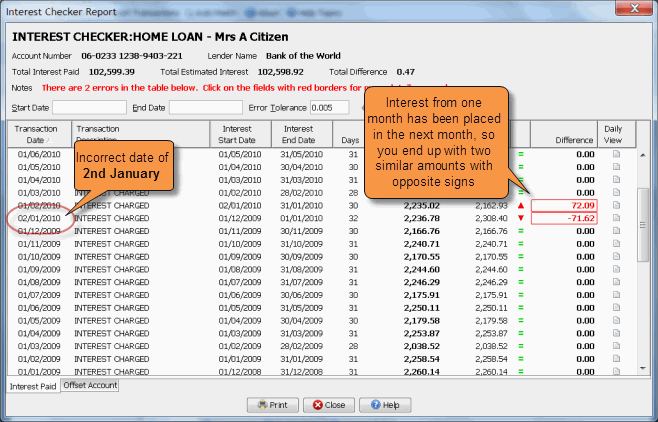### 9. Other Interest Errors

If you have worked through this help topic and you still have an error in the interest checker report then you should:

1. Check that you have correctly entered all dates and amounts for the transactions in the interest date range with the error. For example, if the interest transaction has a period of 1 January 2007 to 31 January 2007 then check all the transaction in this period match the information on your statements.
2. Check that the opening balances on your statements are correct, in particular those that contain the interest transaction with the error.

### 10. Missing or Incorrect Information

If any of the information required to calculate the interest estimates is missing, the estimated interest column will be left empty and an error will be displayed under the in Interest Paid column. Clicking on the error in the report will display a window explaining what information is missing or incorrect.Example - Missing Interest Rate

The interest checker report needs at least one interest rate to be set up on the account before it can estimate the interest. If you run the report before you set up the interest rate every entry will be flagged as a Missing or Incomplete Information and you will be asked to set up an interest rate.

Example - Incorrect Statement Information

Home Loan Manager Pro will not be able to calculate estimated interest amounts for an interest transaction if there are "holes" in the interest period (days missing between statements) or if the opening and closing balances between statements don't match.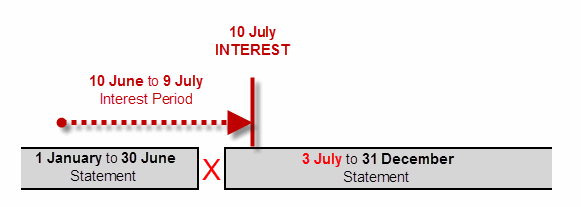For example, if you accidentally create a statement with a start date of the 3rd of July (instead of 1st of July) then there would be 2 days missing in the interest period and the interest can be not be calculated for the 10th of July.

Tip - Run the balance checker report for more information on fixing statement errors.

Example - Partial History

When you first start using Home Loan Manager Pro you may decide that you only wish to enter the most recent statements for your loans. While the software is designed to handle this, it won't be able to check the first interest transaction you enter, as it won't have all the balance changes.For example, if you were to start entering your statements from the 1st of July, then Home Loan Manager Pro wouldn't be able to estimate the interest for the 10th of July, as it wouldn't have a complete list of all the balance changes. Interest transactions after the 10th of July would be calculated correctly, and if at a later date you were to enter the older statements it would go back and calculate an estimate for the 10th of July.

### 11. Interest Rounding Errors

Tip - If there is a very small difference between the estimate and the interest paid, then it could be due to different rounding techniques.

There are many different ways to round the numbers in financial calculations, including rounding up, rounding down, no rounding, rounding to the nearest currency unit, rounding daily, rounding monthly, etc.

In order to maintain the most accurate calculation possible, Home Loan Manager Pro:

1. Does not round any figures during internal calculations.
2. Rounds the final estimated figures to the number of decimal places you have selected under the user Options.

If your loan provider uses a different rounding technique, then the interest checker and refund calculator reports will display a slight difference on most of your interest transactions.

If you decide the errors on the report are due to rounding, and you want to remove them from the report, you can set the Manual Estimate Adjustment amount on each interest transactions to the reported difference. This will ensure they do not appear as errors the next time you run the interest checker and refund calculator report.

Tip - You can edit transactions from within the interest checker report by double-clicking on them.